## Want to keep learning?

This content is taken from the Coventry University's online course, Get ready for a Masters in Data Science and AI. Join the course to learn more.
2.10

# Basic plotting

We often communicate complex information through pictures, diagrams, maps, plots and infographics. When starting to analyse a dataset, simple graphical plots are useful to sanity check data, look for patterns and relationships, and compare different groups.

Exploratory data analysis (EDA) is described by Tukey (1977) as detective work; assembling clues and evidence relevant to solving the case (question). It involves both visualisation of data in various graphical plots and looking at summary statistics such as range, mean, quartiles and correlation coefficient. The aim is to discover patterns and relationships within a dataset so that these can be investigated more deeply by further data analysis.

A graphical plot gives a visual summary of some part of a dataset. In this step, we will consider structured data, such as a table of rows and columns (like a spreadsheet), and how to build some simple graphical plots using the fantastic Matplotlib library for Python (which is already installed with Jupyter Notebook).

## Structured data and variables

When looking at structured data, a row in the table corresponds to a case (also called a record, example, instance or observation) and a column corresponds to a variable (also called a feature, attribute, input or predictor).

When it comes to variables, it is essential to distinguish between categorical variables (names or labels) and quantitative variables (numerical values with magnitude) to ensure we plot the correct type of plot for that variable. For example, in crime data, ‘type of offence’ (criminal damage, common assault, etc) would be a categorical variable, whereas in health data, the ‘resting heart rate’ of an adult would be a quantitative variable.

## Bar graph

A bar graph (or bar chart) is useful for plotting a single categorical variable. For example, we can plot a bar graph (using plt.bar) showing the population of countries using data from Countries in the world by population (2020).

import matplotlib.pyplot as plt
country = ['China', 'India', 'United Sates', 'Indonesia', 'Pakistan', 'Brazil']
population = [1439323776, 1380004385, 331002651, 273523615, 220892340, 212559417]
plt.bar(country,population)
plt.title('Population of Countries (2020)')
plt.xlabel('Country')
plt.ylabel('Population')
plt.show()


The bar graph produced by the Python code above looks like the image below. Note that the ‘1e9’ at the top of the population axis indicates that the values on that axis are in units of $$1\times10^9$$ which is 1 billion. Notice the presence of the title and axis labels.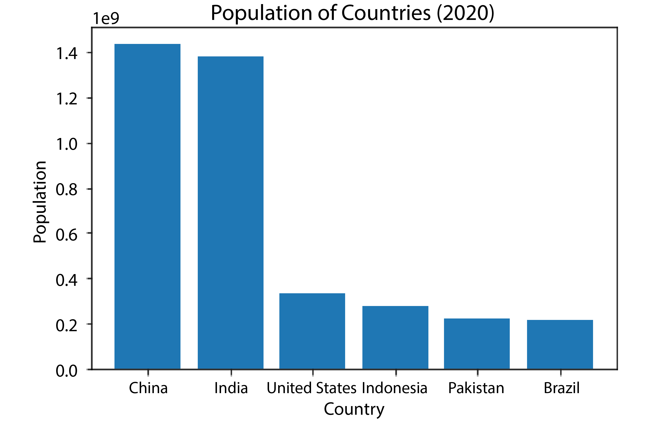(Population of Countries, 2020)

If we replace plt.bar with plt.barh we get a nice horizontal bar graph instead, as in the image below. Try this for yourself.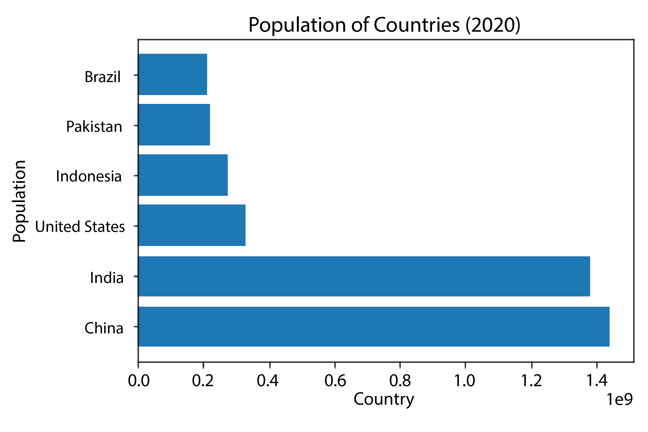(Population of Countries, 2020)

## Line graph

A line graph is useful for plotting a quantitative variable that changes over time. For example, we can plot a line graph (using plt.plot) showing the population of the United Kingdom using data from the Office for National Statistics.

import matplotlib.pyplot as plt
year = [1851, 1861, 1871, 1881, 1891,
1901, 1911, 1921, 1931, 1941,
1951, 1961, 1971, 1981, 1991,
2001, 2011]
population = [ 27368800, 28917900, 31484700, 34934500, 37802400,
41538200, 42189800, 43904100, 46073600, 44870400,
50286900, 52807400, 55928000, 56357500, 57438700,
59113016, 63285145 ]
plt.plot(year, population)
plt.title('Population of United Kingdom')
plt.xlabel('Year')
plt.ylabel('Population')
plt.show()


The line graph produced by the Python code above looks like the image below.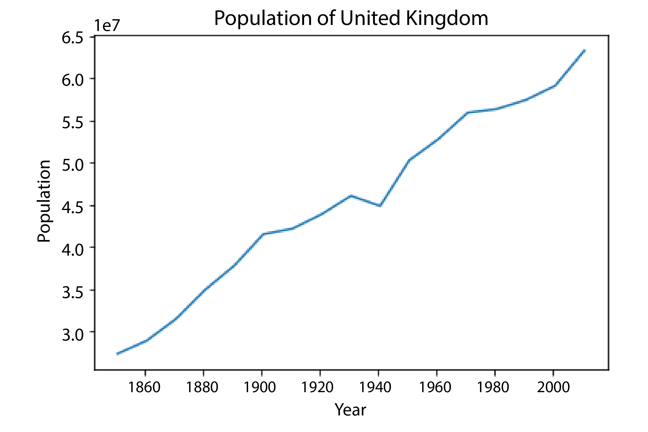## Boxplot

A boxplot is useful for plotting a single quantitative variable. It shows the five-number summary of a dataset visually. The five numbers are the minimum, lower quartile, median, upper quartile and maximum.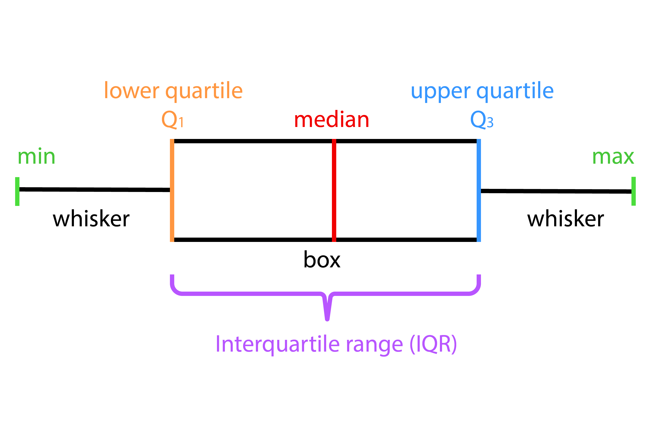For example, using the dataset for number of daily births in the USA (2000-2014) used in a previous step, we could write the following Python code to plot a boxplot (using plt.boxplot) of the data.

import pandas as pd
url = 'https://raw.githubusercontent.com/fivethirtyeight/data/master/births/US_births_2000-2014_SSA.csv'
plt.boxplot(mydata.births, vert=False)
plt.title('Distribution of Number of Daily Births in the USA (2000-2014)')
plt.xlabel('Number of Births')
plt.show()


The boxplot produced by the Python code above looks like the image below. Notice the minimum daily count (just below 6000), the maximum daily count (just above 16000), and the median (approximately 12500) represented by the orange bar.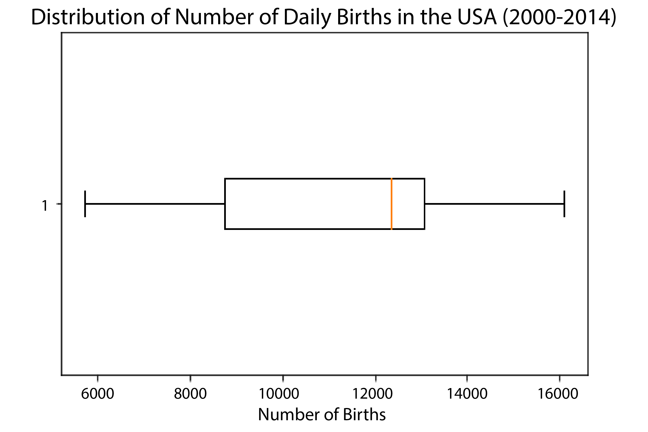Boxplots are especially useful for comparing the distribution of two datasets.

## Scatterplot

A scatterplot is useful for investigating the relationship between two quantitative variables. For example, the following Python code plots a scatterplot (using plt.scatter) of a dataset showing ice cream sales and temperature.

temperature = [14.2, 16.4, 11.9, 15.2, 18.5, 22.1, 19.4, 25.1, 23.4, 18.1, 22.6, 17.2]
icecreamsales = [215, 325, 185, 332, 406, 522, 412, 614, 544, 421, 445, 408]
plt.scatter(temperature, icecreamsales)
plt.title('Ice cream sales vs Temperature')
plt.xlabel('Temperature (degrees C'))
plt.ylabel('Ice cream sales')
plt.show()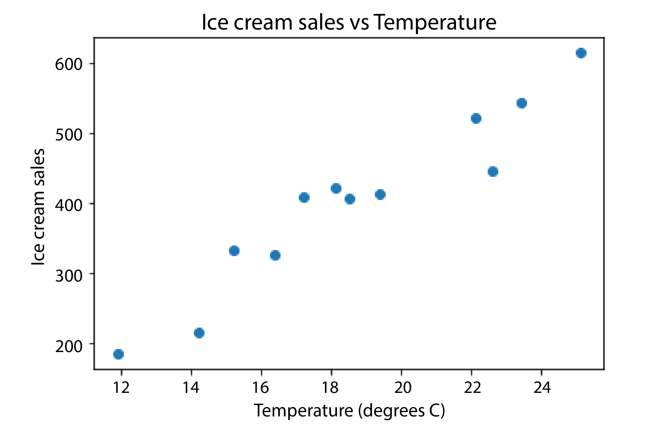## A bit of cartoon fun

You might have seen the xkcd webcomics. They often include graphs as part of the cartoon.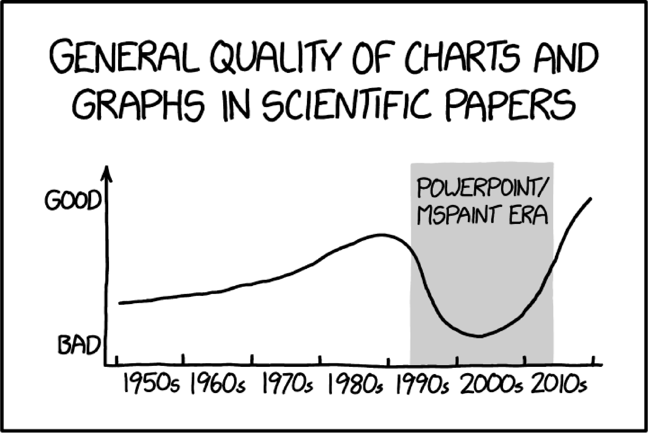Scientific Paper Graph Quality © xkcd, CC BY-NC 2.5

Well, as a bit of fun, adding the line below in the Python code for any of the plots mentioned above, before the first line starting with plt, gives a cartoon version of the plot in the style of xkcd.

plt.xkcd()


For example, the scatterplot above becomes: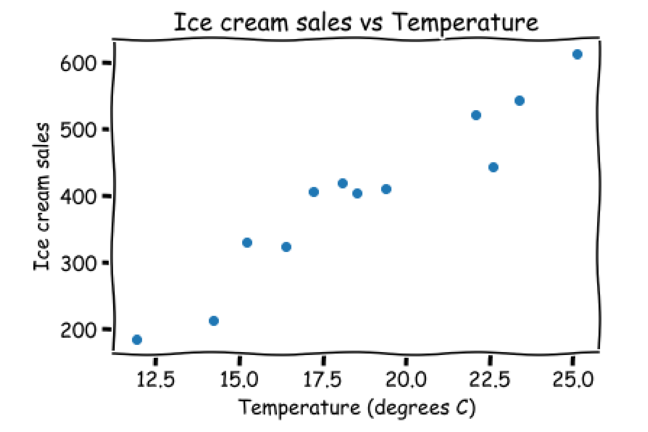The Matplotlib library in Python provides a whole range of different graphical plots. Don’t forget to add a title and axis labels.

Yordanov, V. (2018, July 22). Data science with Python: Intro to data visualization with Matplotlib. Towards Data Science. https://towardsdatascience.com/data-science-with-python-intro-to-data-visualization-and-matplotlib-5f799b7c6d82

Prabhakaran, S. (n.d.). Top 50 matplotlib visualizations - the master plots (with full Python code). Machine Learning Plus. https://www.machinelearningplus.com/plots/top-50-matplotlib-visualizations-the-master-plots-python/

Maths is Fun. (n.d.). Correlation. https://www.mathsisfun.com/data/correlation.html

Office for National Statistics. (2015). UK population estimates 1851 to 2014. https://www.ons.gov.uk/peoplepopulationandcommunity/populationandmigration/populationestimates/adhocs/004356ukpopulationestimates1851to2014

Tukey, J. (1977). Exploratory data analysis. Pearson.

WorldOmetre. (2020). Countries in the world by population (2020). https://www.worldometers.info/world-population/population-by-country/

xkcd. (n.d.). Scientific paper graph quality. https://xkcd.com/1945/